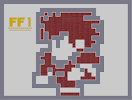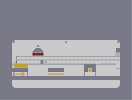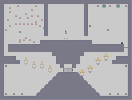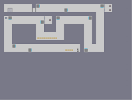### FF1 FighterHover over the thumbnail for a full-size version.

Author dav34 author:dav34 n-art unrated 2006-12-22 2 more votes required for a rating. \$FF1 Fighter#dav34#none#00000000000000000000000000000000000000000000000000000000000000000000000000000000000000000000000000000000000000000000000000000000000000000000000000000000000000000000000000000000001111000011111111001111110010001100100010110100000100010100011101000000001000110000001110000101110001000000100001100011100011000001110001101001110100000110000011010000001000001000000100000000010000000000010000000000100000000001110000000001000000000010010001100010000000000000010011110101000000010000000100001010000000100000001000011101001011000100010000001011011111001111100000001111110001111000000000000000000000000000000000000000000000000000000000000000000000000000000000000000000000000000000000000000000000000000000000000000000000000|12^246,438!12^252,438!12^258,438!12^258,444!12^252,444!12^246,444!12^246,450!12^252,450!12^258,450!12^270,438!12^276,438!12^282,438!12^282,444!12^276,444!12^270,444!12^270,450!12^276,450!12^282,450!12^318,438!12^324,438!12^330,438!12^330,444!12^330,450!12^324,450!12^318,450!12^318,444!12^324,444!12^318,414!12^324,414!12^330,414!12^330,420!12^324,420!12^318,420!12^318,426!12^324,426!12^330,426!12^342,414!12^348,414!12^354,414!12^354,420!12^348,420!12^342,420!12^342,426!12^348,426!12^354,426!12^438,510!12^444,510!12^450,510!12^450,516!12^444,516!12^438,516!12^438,522!12^444,522!12^450,522!12^462,558!12^468,558!12^474,558!12^474,564!12^468,564!12^462,564!12^462,570!12^468,570!12^474,570!12^450,558!12^444,558!12^438,558!12^438,564!12^444,564!12^450,564!12^450,570!12^438,570!12^444,570!12^426,570!12^420,570!12^414,570!12^414,564!12^420,564!12^426,564!12^426,558!12^420,558!12^414,558!12^402,558!12^390,558!12^396,558!12^390,564!12^396,564!12^402,564!12^402,570!12^396,570!12^390,570!12^378,558!12^372,558!12^366,558!12^366,564!12^372,564!12^378,564!12^378,570!12^372,570!12^366,570!12^366,510!12^372,510!12^378,510!12^378,516!12^372,516!12^366,516!12^366,522!12^372,522!12^378,522!12^390,510!12^390,510!12^390,516!12^390,522!12^396,522!12^402,522!12^402,516!12^396,516!12^396,510!12^402,510!12^414,510!12^414,516!12^414,522!12^420,522!12^420,516!12^426,516!12^426,522!12^426,510!12^420,510!12^438,486!12^444,486!12^450,486!12^450,492!12^444,492!12^438,492!12^438,498!12^444,498!12^450,498!12^426,486!12^420,486!12^414,486!12^414,492!12^420,492!12^426,492!12^426,498!12^420,498!12^414,498!12^402,486!12^396,486!12^390,486!12^390,492!12^396,492!12^402,492!12^402,498!12^396,498!12^390,498!12^378,486!12^372,486!12^366,486!12^366,492!12^372,492!12^378,492!12^378,498!12^372,498!12^366,498!12^354,486!12^348,486!12^342,486!12^342,492!12^348,492!12^354,492!12^354,498!12^348,498!12^342,498!12^342,462!12^348,462!12^354,462!12^354,468!12^354,474!12^348,474!12^342,474!12^342,468!12^348,468!12^366,462!12^372,462!12^378,462!12^378,468!12^372,468!12^366,468!12^366,474!12^372,474!12^378,474!12^390,462!12^396,462!12^402,462!12^402,468!12^396,468!12^390,468!12^390,474!12^396,474!12^402,474!12^384,462!12^384,468!12^384,474!12^384,474!12^384,480!12^378,480!12^372,480!12^366,480!12^360,480!12^354,480!12^342,480!12^318,432!12^324,432!12^330,432!12^336,414!12^336,426!12^336,420!12^264,438!12^264,444!12^264,450!12^348,480!12^360,462!12^360,474!12^360,474!12^360,468!12^360,486!12^360,492!12^360,498!12^384,486!12^384,492!12^384,498!12^366,504!12^372,504!12^378,504!12^384,504!12^390,504!12^396,504!12^402,504!12^408,504!12^408,498!12^408,492!12^408,486!12^402,480!12^390,480!12^396,480!12^432,492!12^432,492!12^432,486!12^432,498!12^438,504!12^444,504!12^450,504!12^450,498!12^432,504!12^414,504!12^420,504!12^426,504!12^432,510!12^432,516!12^432,522!12^408,522!12^408,516!12^408,510!12^384,510!12^384,516!12^384,522!12^384,558!12^384,564!12^384,570!12^408,558!12^408,564!12^408,570!12^426,558!12^432,558!12^432,564!12^432,570!12^456,558!12^456,564!12^456,570!12^390,456!12^396,456!12^402,456!12^402,450!12^396,450!12^390,450!12^390,444!12^396,444!12^402,444!12^396,438!12^402,438!12^390,438!12^486,462!12^486,468!12^486,474!12^450,402!12^450,396!12^450,390!12^444,390!12^438,390!12^432,396!12^438,396!12^444,396!12^432,390!12^444,402!12^438,402!12^492,462!12^492,468!12^492,474!12^498,462!12^498,468!12^498,474!12^504,462!12^504,474!12^504,468!12^510,414!12^510,420!12^510,426!12^510,432!12^510,438!12^510,450!12^510,456!12^510,444!12^510,456!12^510,462!12^510,468!12^510,468!12^510,474!12^516,414!12^516,426!12^516,426!12^516,420!12^516,438!12^516,450!12^516,432!12^516,444!12^516,456!12^516,462!12^516,474!12^516,468!12^522,414!12^522,420!12^522,432!12^522,438!12^522,444!12^522,450!12^522,456!12^522,468!12^522,462!12^522,474!12^522,432!12^522,432!12^522,432!12^528,438!12^528,438!12^528,444!12^528,450!12^528,456!12^528,462!12^528,468!12^528,474!12^528,474!12^534,474!12^534,468!12^534,468!12^534,462!12^534,456!12^534,456!12^534,450!12^540,450!12^534,438!12^534,438!12^534,450!12^534,444!12^546,444!12^546,438!12^540,438!12^540,438!12^540,444!12^546,444!12^546,438!12^546,444!12^552,462!12^552,468!12^546,450!12^546,450!12^546,456!12^546,456!12^546,462!12^540,462!12^540,462!12^540,456!12^540,456!12^540,462!12^540,468!12^540,468!12^540,474!12^546,468!12^546,462!12^546,474!12^546,474!12^552,474!12^552,474!12^552,474!12^558,474!12^564,474!12^570,474!12^570,474!12^570,462!12^570,462!12^570,468!12^558,462!12^564,462!12^564,468!12^558,468!12^480,378!12^486,378!12^474,378!12^468,378!12^462,378!12^462,372!12^462,366!12^462,360!12^462,354!12^462,348!12^462,342!12^468,342!12^474,342!12^480,342!12^486,342!12^486,336!12^486,330!12^486,324!12^492,318!12^486,318!12^498,318!12^498,330!12^498,324!12^492,324!12^492,330!12^498,330!12^498,336!12^492,336!12^492,342!12^498,342!12^426,426!12^426,414!12^426,420!12^420,408!12^426,402!12^426,402!12^426,396!12^420,384!12^426,378!12^426,372!12^426,366!12^426,366!12^420,366!12^414,366!12^426,402!12^426,408!12^426,408!12^426,390!12^426,384!12^426,378!12^414,372!12^414,372!12^426,378!12^420,378!12^414,372!12^420,372!12^414,378!12^414,378!12^414,390!12^414,396!12^414,396!12^414,396!12^414,384!12^414,396!12^420,396!12^414,402!12^414,408!12^414,414!12^414,414!12^414,420!12^414,420!12^414,426!12^420,426!12^414,408!12^426,390!12^420,390!12^420,396!12^420,402!12^420,408!12^420,414!12^420,420!12^408,426!12^408,420!12^408,414!12^408,408!12^408,402!12^408,396!12^408,390!12^402,390!12^396,390!12^390,390!12^390,396!12^396,402!12^402,396!12^396,396!12^390,402!12^402,402!12^390,414!12^390,402!12^390,408!12^396,408!12^402,408!12^402,414!12^396,414!12^402,420!12^396,420!12^390,420!12^384,420!12^378,420!12^366,420!12^360,420!12^372,420!12^360,414!12^366,414!12^372,414!12^378,414!12^384,414!12^402,426!12^396,426!12^384,426!12^378,426!12^366,426!12^360,426!12^360,426!12^366,426!12^372,426!12^378,426!12^390,426!12^402,426!12^402,432!12^396,432!12^390,432!12^432,402!12^522,426!12^498,378!12^492,378!12^504,378!12^510,378!12^516,378!12^522,378!12^528,378!12^534,378!12^540,378!12^546,378!12^552,378!12^558,378!12^564,378!12^570,378!12^576,378!12^582,378!12^588,378!12^594,378!12^594,372!12^594,366!12^594,360!12^594,354!12^594,342!12^594,348!12^588,342!12^582,342!12^576,342!12^570,342!12^570,336!12^570,330!12^570,330!12^570,324!12^570,318!12^564,318!12^558,318!12^546,318!12^552,318!12^540,318!12^534,318!12^522,318!12^528,318!12^516,318!12^504,318!12^510,318!12^504,324!12^510,324!12^516,324!12^516,324!12^528,330!12^534,324!12^540,324!12^540,324!12^546,336!12^546,348!12^516,348!12^510,360!12^492,360!12^486,366!12^480,354!12^468,348!12^468,348!12^480,348!12^480,348!12^540,360!12^540,360!12^540,360!12^540,360!12^540,360!12^546,354!12^540,348!12^540,348!12^540,336!12^540,330!12^558,330!12^546,324!12^552,324!12^558,324!12^564,324!12^564,330!12^558,330!12^546,330!12^546,330!12^540,330!12^534,330!12^528,330!12^528,324!12^522,324!12^552,330!12^534,324!12^528,330!12^528,330!12^522,330!12^522,330!12^522,330!12^510,330!12^510,330!12^504,336!12^522,330!12^516,330!12^504,330!12^504,336!12^510,336!12^510,336!12^510,336!12^516,336!12^516,336!12^522,336!12^522,336!12^522,336!12^528,336!12^528,336!12^534,336!12^552,336!12^558,336!12^564,336!12^558,342!12^564,342!12^552,342!12^546,342!12^540,342!12^534,342!12^528,342!12^516,342!12^510,342!12^522,342!12^504,342!12^474,348!12^486,348!12^492,348!12^498,348!12^504,348!12^510,348!12^522,348!12^528,348!12^534,348!12^546,348!12^552,348!12^558,348!12^564,348!12^570,348!12^576,348!12^582,348!12^588,348!12^588,354!12^582,354!12^576,354!12^570,354!12^564,354!12^558,354!12^552,354!12^540,354!12^534,354!12^528,354!12^522,354!12^516,354!12^510,354!12^504,354!12^498,354!12^492,354!12^486,354!12^474,354!12^468,354!12^468,360!12^474,360!12^480,360!12^486,354!12^486,360!12^498,360!12^504,360!12^516,360!12^522,360!12^528,360!12^534,360!12^546,360!12^552,360!12^558,360!12^564,360!12^570,360!12^576,360!12^582,360!12^582,360!12^588,360!12^588,366!12^588,366!12^582,366!12^582,366!12^576,366!12^558,366!12^564,366!12^564,366!12^564,366!12^570,366!12^558,372!12^540,366!12^540,366!12^546,366!12^552,366!12^546,366!12^534,366!12^528,366!12^516,366!12^510,366!12^498,366!12^498,366!12^486,366!12^480,366!12^474,366!12^462,360!12^468,360!12^468,372!12^480,366!12^468,360!12^468,366!12^474,366!12^468,366!12^480,366!12^498,366!12^504,366!12^516,366!12^522,366!12^522,366!12^510,366!12^504,366!12^492,366!12^468,372!12^474,372!12^480,372!12^486,372!12^486,372!12^492,372!12^498,372!12^504,372!12^510,372!12^522,372!12^522,372!12^534,372!12^546,372!12^552,372!12^570,372!12^576,372!12^588,372!12^588,372!12^576,372!12^558,372!12^564,372!12^564,372!12^582,372!12^540,372!12^528,372!12^516,372!12^498,306!12^498,300!12^498,294!12^492,294!12^480,294!12^486,294!12^480,300!12^486,300!12^486,306!12^492,300!12^492,306!12^480,306!12^474,306!12^474,300!12^474,294!12^468,294!12^468,300!12^468,306!12^462,306!12^462,300!12^462,294!12^510,270!12^510,276!12^510,282!12^516,270!12^516,276!12^516,282!12^522,270!12^522,276!12^522,282!12^528,270!12^528,276!12^528,282!12^528,282!12^534,276!12^534,270!12^534,282!12^540,282!12^540,276!12^540,270!12^546,270!12^546,282!12^546,276!12^546,264!12^540,264!12^534,264!12^528,264!12^522,264!12^516,264!12^510,264!12^510,258!12^516,258!12^522,258!12^528,258!12^540,258!12^546,258!12^534,258!12^552,258!12^558,258!12^564,258!12^570,258!12^570,252!12^570,246!12^564,246!12^564,252!12^558,252!12^558,246!12^546,246!12^552,252!12^552,246!12^546,252!12^540,252!12^540,246!12^534,246!12^534,252!12^534,252!12^528,252!12^534,246!12^528,246!12^522,252!12^522,246!12^516,246!12^516,252!12^510,252!12^510,246!12^510,240!12^516,240!12^522,240!12^528,240!12^534,240!12^540,240!12^546,240!12^546,234!12^540,234!12^528,234!12^534,234!12^522,234!12^516,234!12^510,234!12^510,228!12^510,228!12^516,228!12^522,228!12^528,228!12^534,228!12^540,228!12^546,228!12^540,222!12^546,222!12^534,222!12^528,222!12^522,222!12^516,222!12^510,222!12^510,216!12^516,216!12^522,216!12^528,216!12^534,216!12^546,216!12^540,216!12^546,210!12^540,210!12^534,210!12^528,210!12^516,210!12^510,210!12^516,210!12^522,210!12^552,210!12^558,210!12^564,210!12^570,210!12^576,210!12^582,210!12^588,210!12^594,210!12^594,204!12^594,198!12^588,198!12^588,204!12^582,204!12^582,198!12^576,198!12^576,204!12^570,204!12^570,198!12^570,192!12^564,192!12^564,198!12^564,198!12^564,204!12^558,204!12^558,198!12^558,192!12^552,192!12^552,198!12^552,204!12^546,204!12^546,198!12^546,192!12^534,192!12^534,204!12^540,204!12^540,198!12^540,192!12^534,198!12^528,192!12^528,204!12^528,198!12^522,192!12^522,198!12^522,204!12^516,192!12^510,198!12^516,204!12^516,198!12^510,192!12^510,204!12^570,186!12^570,180!12^570,174!12^564,174!12^558,174!12^552,174!12^552,180!12^558,180!12^564,180!12^564,186!12^558,186!12^552,186!12^546,186!12^534,186!12^528,186!12^534,186!12^540,186!12^546,180!12^546,174!12^546,174!12^540,174!12^540,180!12^534,180!12^534,174!12^528,174!12^528,180!12^528,180!12^522,186!12^510,186!12^516,186!12^522,180!12^522,174!12^516,174!12^510,180!12^516,180!12^510,174!12^546,168!12^546,156!12^546,168!12^546,168!12^546,162!12^546,150!12^540,150!12^534,150!12^522,150!12^528,150!12^516,150!12^510,150!12^510,156!12^510,162!12^510,168!12^516,156!12^522,156!12^528,156!12^528,156!12^534,156!12^534,156!12^540,156!12^540,156!12^540,156!12^540,162!12^534,162!12^522,162!12^522,162!12^516,162!12^510,162!12^522,162!12^534,162!12^534,162!12^546,174!12^534,168!12^534,168!12^522,162!12^528,162!12^528,168!12^534,168!12^540,168!12^528,168!12^522,162!12^522,168!12^516,168!12^510,144!12^510,138!12^510,132!12^510,126!12^516,126!12^516,132!12^516,138!12^516,144!12^516,126!12^522,126!12^522,132!12^522,138!12^522,144!12^528,126!12^528,132!12^528,138!12^528,144!12^534,126!12^534,138!12^534,132!12^534,144!12^540,126!12^540,132!12^540,138!12^540,144!12^546,144!12^546,138!12^546,132!12^546,126!12^552,126!12^552,132!12^552,138!12^558,138!12^558,132!12^558,126!12^564,126!12^564,132!12^564,138!12^570,138!12^570,138!12^570,132!12^570,126!12^576,126!12^576,132!12^576,138!12^582,138!12^582,132!12^582,126!12^588,126!12^588,132!12^588,138!12^594,138!12^594,132!12^594,126!12^546,90!12^546,84!12^546,78!12^540,78!12^540,84!12^540,90!12^534,90!12^534,84!12^534,78!12^528,78!12^528,84!12^528,90!12^522,90!12^522,84!12^522,78!12^516,84!12^516,90!12^516,78!12^510,78!12^510,84!12^510,90!12^504,90!12^504,84!12^504,78!12^498,78!12^492,90!12^498,84!12^498,90!12^492,84!12^492,78!12^480,78!12^486,78!12^486,90!12^486,84!12^480,84!12^480,90!12^474,78!12^474,84!12^474,90!12^468,84!12^468,90!12^468,84!12^468,78!12^462,84!12^462,78!12^462,90!12^456,90!12^456,84!12^456,78!12^456,78!12^450,78!12^450,84!12^450,90!12^444,90!12^444,84!12^444,78!12^438,78!12^438,90!12^438,84!12^432,90!12^432,84!12^432,84!12^432,78!12^426,84!12^426,78!12^426,90!12^420,90!12^420,78!12^420,84!12^414,78!12^414,84!12^414,90!12^408,90!12^408,84!12^408,78!12^402,78!12^402,84!12^402,90!12^396,90!12^396,84!12^396,78!12^390,84!12^390,90!12^390,78!12^384,78!12^384,90!12^384,84!12^378,84!12^378,90!12^378,78!12^372,78!12^372,84!12^372,90!12^366,90!12^366,84!12^366,78!12^498,102!12^492,102!12^486,102!12^474,102!12^480,102!12^468,102!12^456,102!12^456,102!12^456,102!12^462,102!12^450,102!12^444,102!12^438,102!12^432,102!12^426,102!12^420,102!12^414,102!12^408,96!12^408,102!12^402,102!12^498,96!12^486,96!12^486,96!12^486,96!12^492,96!12^474,96!12^474,96!12^468,96!12^468,96!12^462,96!12^474,96!12^480,96!12^468,90!12^450,90!12^444,90!12^432,96!12^426,96!12^444,96!12^450,96!12^456,96!12^456,96!12^450,96!12^438,96!12^432,96!12^426,90!12^414,96!12^414,90!12^366,96!12^372,96!12^384,96!12^378,96!12^396,96!12^420,96!12^402,96!12^390,90!12^390,96!12^396,102!12^390,102!12^390,102!12^384,102!12^378,102!12^372,102!12^366,102!12^366,108!12^372,108!12^378,108!12^384,108!12^498,108!12^486,108!12^480,108!12^486,108!12^486,108!12^492,108!12^474,108!12^462,108!12^468,108!12^456,108!12^444,108!12^444,108!12^438,108!12^438,108!12^426,102!12^420,102!12^414,102!12^414,108!12^408,108!12^396,108!12^390,108!12^384,108!12^390,108!12^402,108!12^414,108!12^426,108!12^432,108!12^438,108!12^450,108!12^450,108!12^426,108!12^420,108!12^498,114!12^492,114!12^486,114!12^480,114!12^474,114!12^462,114!12^468,120!12^468,114!12^456,114!12^450,114!12^438,114!12^426,114!12^438,114!12^444,114!12^432,114!12^426,114!12^426,114!12^420,114!12^420,114!12^408,114!12^402,114!12^390,108!12^384,114!12^384,114!12^366,114!12^366,114!12^366,114!12^378,114!12^384,114!12^396,114!12^408,114!12^408,114!12^408,114!12^408,114!12^414,114!12^414,114!12^408,114!12^402,114!12^390,114!12^390,114!12^384,114!12^366,114!12^372,108!12^366,120!12^372,120!12^372,120!12^378,120!12^378,120!12^384,120!12^384,120!12^396,120!12^396,120!12^372,114!12^390,120!12^402,120!12^408,120!12^420,126!12^414,120!12^408,120!12^414,120!12^426,120!12^432,120!12^444,120!12^456,120!12^468,120!12^492,120!12^492,120!12^498,120!12^486,120!12^486,120!12^480,120!12^474,120!12^462,120!12^450,120!12^450,120!12^450,120!12^438,120!12^432,120!12^420,120!12^366,126!12^372,126!12^372,126!12^378,126!12^384,126!12^384,126!12^384,126!12^390,126!12^396,126!12^396,126!12^402,126!12^408,126!12^408,126!12^414,126!12^420,126!12^432,126!12^438,126!12^444,126!12^450,126!12^456,126!12^468,126!12^480,126!12^492,126!12^498,126!12^486,126!12^474,126!12^456,126!12^462,126!12^420,126!12^426,126!12^504,126!12^498,132!12^504,138!12^504,144!12^504,144!12^504,150!12^504,132!12^504,156!12^504,162!12^504,162!12^504,168!12^504,174!12^504,180!12^504,186!12^510,192!12^510,192!12^510,198!12^504,192!12^504,198!12^504,198!12^504,204!12^504,210!12^504,222!12^504,228!12^504,228!12^498,210!12^504,222!12^504,216!12^498,138!12^504,234!12^492,234!12^498,234!12^498,228!12^498,222!12^498,216!12^498,210!12^498,204!12^498,204!12^498,198!12^498,192!12^498,192!12^498,186!12^498,180!12^498,174!12^498,174!12^498,174!12^498,168!12^498,168!12^498,162!12^498,156!12^498,156!12^498,150!12^498,144!12^492,228!12^492,228!12^492,222!12^492,222!12^492,216!12^492,210!12^492,204!12^492,198!12^492,198!12^492,192!12^492,180!12^492,180!12^492,174!12^492,168!12^492,168!12^492,156!12^492,144!12^492,132!12^492,126!12^492,132!12^492,138!12^492,150!12^492,162!12^492,168!12^492,180!12^492,186!12^492,186!12^486,234!12^486,234!12^486,234!12^486,234!12^480,222!12^480,216!12^480,210!12^486,228!12^486,228!12^486,222!12^486,222!12^486,216!12^486,216!12^486,210!12^486,210!12^486,204!12^492,186!12^492,186!12^486,204!12^486,204!12^486,204!12^486,204!12^486,204!12^486,204!12^486,204!12^486,204!12^486,192!12^480,162!12^480,162!12^480,162!12^480,162!12^480,162!12^480,138!12^480,138!12^480,138!12^480,138!12^486,186!12^486,186!12^486,180!12^480,174!12^486,162!12^480,150!12^486,132!12^492,126!12^492,126!12^486,138!12^486,150!12^480,180!12^480,198!12^486,180!12^480,150!12^480,138!12^480,144!12^486,168!12^486,174!12^480,138!12^486,150!12^486,156!12^486,138!12^480,150!12^480,156!12^480,156!12^480,162!12^480,180!12^486,138!12^486,144!12^474,168!12^480,168!12^480,180!12^480,186!12^480,198!12^486,204!12^486,198!12^474,192!12^492,198!12^480,192!12^480,198!12^480,204!12^480,204!12^480,234!12^480,228!12^474,234!12^474,228!12^474,222!12^474,216!12^468,210!12^474,210!12^474,204!12^474,204!12^474,198!12^474,186!12^474,186!12^474,180!12^474,174!12^474,162!12^474,156!12^474,144!12^474,138!12^474,132!12^480,132!12^474,150!12^468,234!12^468,228!12^468,222!12^468,216!12^468,204!12^468,198!12^468,192!12^468,180!12^468,174!12^468,168!12^468,156!12^468,186!12^468,162!12^468,150!12^468,144!12^468,138!12^468,132!12^462,132!12^462,144!12^462,138!12^462,156!12^462,168!12^462,150!12^462,168!12^462,168!12^462,180!12^462,180!12^462,180!12^462,192!12^462,198!12^462,210!12^462,216!12^462,228!12^462,234!12^462,234!12^468,228!12^462,222!12^462,204!12^462,186!12^462,174!12^462,168!12^462,162!12^456,132!12^456,138!12^456,144!12^456,144!12^456,156!12^456,156!12^456,162!12^456,174!12^456,180!12^456,168!12^456,156!12^456,150!12^456,156!12^456,162!12^456,174!12^456,174!12^456,180!12^456,186!12^456,186!12^456,192!12^456,198!12^456,198!12^456,204!12^456,204!12^456,210!12^456,216!12^456,216!12^456,222!12^456,234!12^456,228!12^450,234!12^450,228!12^450,228!12^450,216!12^450,210!12^450,204!12^450,210!12^450,216!12^450,222!12^450,210!12^450,198!12^450,198!12^450,192!12^450,192!12^450,192!12^450,186!12^450,186!12^450,180!12^450,180!12^450,174!12^450,174!12^450,168!12^450,162!12^450,162!12^450,156!12^450,156!12^450,150!12^450,150!12^450,144!12^450,138!12^450,138!12^450,132!12^444,132!12^444,132!12^444,138!12^444,144!12^444,144!12^444,144!12^444,144!12^444,150!12^444,150!12^444,156!12^444,156!12^444,156!12^444,156!12^444,162!12^444,162!12^444,168!12^444,174!12^444,180!12^444,180!12^444,186!12^444,186!12^444,192!12^444,192!12^444,198!12^444,198!12^444,204!12^444,204!12^444,210!12^444,210!12^444,216!12^444,216!12^444,222!12^444,234!12^444,228!12^438,234!12^438,228!12^438,222!12^438,222!12^438,216!12^438,216!12^438,210!12^438,210!12^438,210!12^438,204!12^438,204!12^438,204!12^438,204!12^438,198!12^438,192!12^438,192!12^438,192!12^438,186!12^438,186!12^438,186!12^438,180!12^438,180!12^438,180!12^438,174!12^438,174!12^438,168!12^438,168!12^438,162!12^438,162!12^438,156!12^438,156!12^438,150!12^438,144!12^438,138!12^438,138!12^438,132!12^432,132!12^432,132!12^432,132!12^432,138!12^432,144!12^432,144!12^432,144!12^432,150!12^432,156!12^432,162!12^432,162!12^432,168!12^432,180!12^432,186!12^432,192!12^432,192!12^432,198!12^432,192!12^432,180!12^432,174!12^432,192!12^438,198!12^432,210!12^432,210!12^432,204!12^432,204!12^432,210!12^432,216!12^432,222!12^432,228!12^432,240!12^432,234!12^426,234!12^426,228!12^420,222!12^426,222!12^426,216!12^426,204!12^426,198!12^426,192!12^426,186!12^426,174!12^432,162!12^432,150!12^426,132!12^426,126!12^426,132!12^426,132!12^426,132!12^426,144!12^426,150!12^420,156!12^420,156!12^420,162!12^426,174!12^426,180!12^426,180!12^426,186!12^426,186!12^426,192!12^426,192!12^426,162!12^426,162!12^426,162!12^426,156!12^426,150!12^426,150!12^426,162!12^426,174!12^426,168!12^426,150!12^426,132!12^426,132!12^426,156!12^432,198!12^426,204!12^426,204!12^426,210!12^414,222!12^420,222!12^420,210!12^420,198!12^420,192!12^420,180!12^420,174!12^420,162!12^420,144!12^420,132!12^426,132!12^426,138!12^420,138!12^420,132!12^420,132!12^420,144!12^420,156!12^420,156!12^420,156!12^420,168!12^420,156!12^420,150!12^420,150!12^426,168!12^426,174!12^420,180!12^420,180!12^420,192!12^420,192!12^420,198!12^420,198!12^420,186!12^420,186!12^420,198!12^420,204!12^420,204!12^420,210!12^420,210!12^420,216!12^420,228!12^420,228!12^420,228!12^420,228!12^420,234!12^420,234!12^414,240!12^426,234!12^420,240!12^426,240!12^414,240!12^414,234!12^414,234!12^414,228!12^414,222!12^414,210!12^414,210!12^414,204!12^414,198!12^414,192!12^414,186!12^414,180!12^414,174!12^414,168!12^414,168!12^414,162!12^414,156!12^414,150!12^414,150!12^408,144!12^408,138!12^414,138!12^414,132!12^414,132!12^414,132!12^414,126!12^414,126!12^408,138!12^408,144!12^420,144!12^420,144!12^414,144!12^414,216!12^414,246!12^420,246!12^432,246!12^426,252!12^414,252!12^420,252!12^426,246!12^432,252!12^432,258!12^426,258!12^414,258!12^420,258!12^438,240!12^444,240!12^450,240!12^444,252!12^444,240!12^432,252!12^444,240!12^444,246!12^450,246!12^438,246!12^438,252!12^450,252!12^450,258!12^444,258!12^438,258!12^438,270!12^444,270!12^450,264!12^444,264!12^438,264!12^450,270!12^450,276!12^450,282!12^444,282!12^438,282!12^438,276!12^444,276!12^408,138!12^408,132!12^408,144!12^408,150!12^408,150!12^408,150!12^408,156!12^408,162!12^408,168!12^408,168!12^408,174!12^408,174!12^408,180!12^408,180!12^408,186!12^402,186!12^402,180!12^402,180!12^402,174!12^402,162!12^402,162!12^402,156!12^402,144!12^402,144!12^402,138!12^402,138!12^402,132!12^402,132!12^402,144!12^402,150!12^402,156!12^402,156!12^402,162!12^402,168!12^402,168!12^396,186!12^396,180!12^396,174!12^390,168!12^390,162!12^396,156!12^396,150!12^396,144!12^396,132!12^396,132!12^396,132!12^396,132!12^402,138!12^396,144!12^396,150!12^396,150!12^396,156!12^390,156!12^396,162!12^396,162!12^396,162!12^396,162!12^396,162!12^396,162!12^396,168!12^396,168!12^390,186!12^390,180!12^390,174!12^390,162!12^390,150!12^396,150!12^396,144!12^396,138!12^396,138!12^396,138!12^390,138!12^390,138!12^390,144!12^390,132!12^390,132!12^366,132!12^366,138!12^366,138!12^366,144!12^366,144!12^366,144!12^366,150!12^366,150!12^366,150!12^366,156!12^366,162!12^366,162!12^366,162!12^366,168!12^366,168!12^366,168!12^384,186!12^378,186!12^366,186!12^372,186!12^366,180!12^366,174!12^372,174!12^372,180!12^372,180!12^378,180!12^378,180!12^384,174!12^384,174!12^378,174!12^384,168!12^378,168!12^378,168!12^372,168!12^384,180!12^372,162!12^372,162!12^372,156!12^378,156!12^372,150!12^372,144!12^372,138!12^372,138!12^372,132!12^378,132!12^384,132!12^384,132!12^384,132!12^384,132!12^378,144!12^384,144!12^384,150!12^378,156!12^384,162!12^384,162!12^384,162!12^384,162!12^384,162!12^384,162!12^378,162!12^384,162!12^384,156!12^384,156!12^384,150!12^384,150!12^378,144!12^384,132!12^384,138!12^384,144!12^384,144!12^378,144!12^378,138!12^378,138!12^378,144!12^378,150!12^360,186!12^354,186!12^354,180!12^354,174!12^354,168!12^354,168!12^354,156!12^354,156!12^354,150!12^354,144!12^354,144!12^354,144!12^360,132!12^360,132!12^354,126!12^354,120!12^360,114!12^360,114!12^360,108!12^360,102!12^354,102!12^360,114!12^360,120!12^360,126!12^360,126!12^360,138!12^360,144!12^360,156!12^360,156!12^360,162!12^360,168!12^360,168!12^360,174!12^360,174!12^360,180!12^360,180!12^360,180!12^360,150!12^354,162!12^354,138!12^354,132!12^354,114!12^354,108!12^348,102!12^348,108!12^348,120!12^348,114!12^348,132!12^348,126!12^348,138!12^348,150!12^348,144!12^348,156!12^348,168!12^348,162!12^348,174!12^348,180!12^348,186!12^354,192!12^354,198!12^354,204!12^354,210!12^348,210!12^348,204!12^348,198!12^348,192!12^342,210!12^336,210!12^342,204!12^336,204!12^342,198!12^336,198!12^336,192!12^342,192!12^342,186!12^336,186!12^330,180!12^336,180!12^342,180!12^342,168!12^336,174!12^342,174!12^342,162!12^330,168!12^336,168!12^336,162!12^342,156!12^336,156!12^336,150!12^342,150!12^342,144!12^336,144!12^336,138!12^342,138!12^342,132!12^336,132!12^336,126!12^342,126!12^342,120!12^336,120!12^336,114!12^342,108!12^342,114!12^336,108!12^336,102!12^342,102!12^330,210!12^330,204!12^330,198!12^330,186!12^330,186!12^330,180!12^330,174!12^330,192!12^330,162!12^330,156!12^330,150!12^330,144!12^330,138!12^330,132!12^330,126!12^330,120!12^330,114!12^330,108!12^330,96!12^330,102!12^330,90!12^330,84!12^330,78!12^324,78!12^318,78!12^318,90!12^324,84!12^318,84!12^324,90!12^324,96!12^324,102!12^324,108!12^324,114!12^324,120!12^324,120!12^324,120!12^324,126!12^324,126!12^324,126!12^324,132!12^324,138!12^324,138!12^324,138!12^324,138!12^324,144!12^324,144!12^330,150!12^330,150!12^330,156!12^330,156!12^324,150!12^324,156!12^324,162!12^324,162!12^324,162!12^324,168!12^324,168!12^324,174!12^324,180!12^324,180!12^324,180!12^324,186!12^324,186!12^324,192!12^324,198!12^324,198!12^324,210!12^324,204!12^318,210!12^318,204!12^318,198!12^318,192!12^318,186!12^318,180!12^318,174!12^318,168!12^318,162!12^318,156!12^318,144!12^318,138!12^318,132!12^324,150!12^318,150!12^318,120!12^318,120!12^318,108!12^318,96!12^318,102!12^318,108!12^318,108!12^318,114!12^318,120!12^324,126!12^318,126!12^312,102!12^312,108!12^312,108!12^312,114!12^312,120!12^312,120!12^312,126!12^312,126!12^312,132!12^312,138!12^312,138!12^312,144!12^312,144!12^312,144!12^312,150!12^312,150!12^312,156!12^312,156!12^312,162!12^312,168!12^312,168!12^312,168!12^312,174!12^312,174!12^312,180!12^312,186!12^312,192!12^312,192!12^312,198!12^312,198!12^312,204!12^312,210!12^306,102!12^306,108!12^306,114!12^306,114!12^306,114!12^306,114!12^300,126!12^300,126!12^306,132!12^306,120!12^306,126!12^306,132!12^306,138!12^306,144!12^306,144!12^306,150!12^306,156!12^306,162!12^306,162!12^306,168!12^306,168!12^306,174!12^306,180!12^306,186!12^306,192!12^306,198!12^306,204!12^306,204!12^306,210!12^306,216!12^306,216!12^306,222!12^306,222!12^306,228!12^306,228!12^306,234!12^300,102!12^300,102!12^300,114!12^300,114!12^300,114!12^300,120!12^300,126!12^300,126!12^300,108!12^300,138!12^300,132!12^300,144!12^300,144!12^300,144!12^300,156!12^300,156!12^300,156!12^300,162!12^300,162!12^300,162!12^300,174!12^300,174!12^300,180!12^300,180!12^300,186!12^300,186!12^300,192!12^300,192!12^300,198!12^300,198!12^300,204!12^300,204!12^300,210!12^300,210!12^300,216!12^300,216!12^300,222!12^300,222!12^300,228!12^300,234!12^300,168!12^300,150!12^294,102!12^294,102!12^294,108!12^294,114!12^294,126!12^294,120!12^294,132!12^294,132!12^294,138!12^294,138!12^294,144!12^294,144!12^294,150!12^294,150!12^294,156!12^294,162!12^294,162!12^294,168!12^294,168!12^294,174!12^294,174!12^294,180!12^294,180!12^294,186!12^294,192!12^294,192!12^294,198!12^294,198!12^294,204!12^294,204!12^294,210!12^294,216!12^294,216!12^294,228!12^294,234!12^294,222!12^288,126!12^288,132!12^288,132!12^288,138!12^288,138!12^288,144!12^288,144!12^288,150!12^288,156!12^288,162!12^288,168!12^288,174!12^288,174!12^288,180!12^288,180!12^288,186!12^288,186!12^282,126!12^282,132!12^282,138!12^282,144!12^282,150!12^282,156!12^282,162!12^282,162!12^282,162!12^282,168!12^282,174!12^282,174!12^282,180!12^282,186!12^282,186!12^276,186!12^276,180!12^276,174!12^276,168!12^276,162!12^276,156!12^276,150!12^276,144!12^276,138!12^276,126!12^276,132!12^270,126!12^264,126!12^258,126!12^252,126!12^246,126!12^246,132!12^246,138!12^252,138!12^258,138!12^264,138!12^270,138!12^270,132!12^264,132!12^258,132!12^252,132!12^270,150!12^270,144!12^270,150!12^270,150!12^270,156!12^270,162!12^270,162!12^270,168!12^270,168!12^270,174!12^270,180!12^270,186!12^270,186!12^270,186!12^270,78!12^270,84!12^270,84!12^270,84!12^276,90!12^270,90!12^282,90!12^282,84!12^282,78!12^276,78!12^276,84!12^246,102!12^246,108!12^246,108!12^246,108!12^258,102!12^258,102!12^258,108!12^258,114!12^258,114!12^252,102!12^252,102!12^252,102!12^252,108!12^252,114!12^252,114!12^246,114!12^246,108!12^246,114!12^246,120!12^246,120!12^252,120!12^252,114!12^258,120!12^258,120!12^258,120!12^246,174!12^246,180!12^246,186!12^246,192!12^246,198!12^252,210!12^252,210!12^252,216!12^246,204!12^246,216!12^246,222!12^246,228!12^246,210!12^246,234!12^252,234!12^258,234!12^258,228!12^258,228!12^258,222!12^258,216!12^258,216!12^258,216!12^258,210!12^258,204!12^258,198!12^258,198!12^258,192!12^258,192!12^258,186!12^258,180!12^258,180!12^258,174!12^252,174!12^252,180!12^246,180!12^246,186!12^246,186!12^246,192!12^246,198!12^252,186!12^252,186!12^252,192!12^252,198!12^252,204!12^252,210!12^252,216!12^252,222!12^252,222!12^252,228!12^270,318!12^270,324!12^270,330!12^270,342!12^270,336!12^264,342!12^258,342!12^252,342!12^246,342!12^246,348!12^246,354!12^252,354!12^252,348!12^258,348!12^258,354!12^270,402!12^270,396!12^270,390!12^276,402!12^282,402!12^294,402!12^288,402!12^300,402!12^312,402!12^306,402!12^318,408!12^318,402!12^324,408!12^324,402!12^330,402!12^330,408!12^306,318!12^306,318!12^306,324!12^306,330!12^282,318!12^276,318!12^288,318!12^294,318!12^300,318!12^300,330!12^300,324!12^288,324!12^294,324!12^294,330!12^288,330!12^282,330!12^282,324!12^276,324!12^276,330!12^282,432!12^276,432!12^270,432!12^276,336!12^282,336!12^288,336!12^300,336!12^306,336!12^294,336!12^306,342!12^300,342!12^294,348!12^288,342!12^294,342!12^282,342!12^276,342!12^270,348!12^264,348!12^276,348!12^282,348!12^294,348!12^300,348!12^306,348!12^288,348!12^306,354!12^300,354!12^294,354!12^288,354!12^282,354!12^276,360!12^276,354!12^264,354!12^270,354!12^270,360!12^282,360!12^288,360!12^300,360!12^306,360!12^294,360!12^306,366!12^300,366!12^294,366!12^288,366!12^282,366!12^276,366!12^270,366!12^270,372!12^276,372!12^282,372!12^288,372!12^294,372!12^300,372!12^306,372!12^306,378!12^300,378!12^294,378!12^282,378!12^276,378!12^288,378!12^270,378!12^270,384!12^276,384!12^288,384!12^294,384!12^300,384!12^306,384!12^282,384!12^276,390!12^282,390!12^294,396!12^288,390!12^294,390!12^300,390!12^306,390!12^312,390!12^318,390!12^324,390!12^330,390!12^330,396!12^324,396!12^318,396!12^318,396!12^312,396!12^306,396!12^300,396!12^294,396!12^288,396!12^282,396!12^276,396!12^264,390!12^258,390!12^252,390!12^246,390!12^246,396!12^258,396!12^264,396!12^252,396!12^246,402!12^252,402!12^264,402!12^258,402!12^282,408!12^276,408!12^270,408!12^270,408!12^264,408!12^258,408!12^252,408!12^246,408!12^246,414!12^252,414!12^258,414!12^264,414!12^276,414!12^282,414!12^270,414!12^282,420!12^276,420!12^270,426!12^270,420!12^264,420!12^258,420!12^252,420!12^246,420!12^246,426!12^264,426!12^258,426!12^252,426!12^276,426!12^282,426!12^264,432!12^258,432!12^252,432!12^246,432!0^60,108!0^60,114!0^60,120!0^60,126!0^60,132!0^60,138!0^60,144!0^60,150!0^60,156!0^66,156!0^72,156!0^66,150!0^72,150!0^72,144!0^66,144!0^72,138!0^66,138!0^66,132!0^72,132!0^72,126!0^66,126!0^66,126!0^72,126!0^72,120!0^66,120!0^66,114!0^72,114!0^72,108!0^66,108!0^78,108!0^84,108!0^90,108!0^96,108!0^96,114!0^90,114!0^84,114!0^78,114!0^78,126!0^84,126!0^90,126!0^90,132!0^84,132!0^78,132!0^108,108!0^108,114!0^108,120!0^108,126!0^108,138!0^108,132!0^108,144!0^108,150!0^108,156!0^114,156!0^114,156!0^114,150!0^120,156!0^120,150!0^120,144!0^120,138!0^120,138!0^120,132!0^120,126!0^120,126!0^120,120!0^120,120!0^120,114!0^120,114!0^120,108!0^114,108!0^114,114!0^114,120!0^114,120!0^114,126!0^114,132!0^114,138!0^114,144!0^126,108!0^132,108!0^138,108!0^144,108!0^144,114!0^138,114!0^132,114!0^126,114!0^126,126!0^132,126!0^138,126!0^138,126!0^138,132!0^132,132!0^132,132!0^126,132!0^168,108!0^174,114!0^174,108!0^180,108!0^180,114!0^174,120!0^180,120!0^180,126!0^174,126!0^174,132!0^180,132!0^180,138!0^180,144!0^180,150!0^180,156!0^174,156!0^174,150!0^174,144!0^174,138!0^54,168!0^66,168!0^78,168!0^90,168!0^102,168!0^114,168!0^126,168!0^138,168!0^150,168!0^162,168!0^174,168!0^186,168!0^60,180!0^72,180!0^84,180!0^96,180!0^108,180!0^120,180!0^132,180!0^144,180!0^156,180!0^168,180!0^180,180# Took me like half an hour 4th map! bewere:takes a while to load in editor

## Other maps by this authorFirst Level =D A Random Map My Third = )

Pages: (0)

### Done b4

http://numa.notdot.net/map/24794

### I

Always rate 5/5 But this gets a 4/5 on the off list... IE i say 4/5 or some other rating but I will always rate 5/5

thx

### nice

took awhile for me to see the picure though
5/5